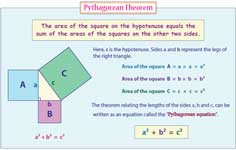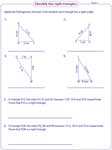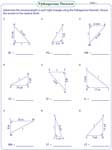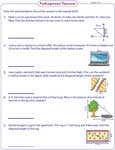1. Worksheets>
2. Math>
3. Geometry>
4. Pythagorean Theorem

# Pythagorean Theorem Worksheets

These worksheets have exercises on finding the leg and hypotenuse of a right triangle using the Pythagorean theorem. Pythagorean triple charts with exercises are provided here. Word problems on real time application are available. Moreover, descriptive charts on the application of the theorem in different shapes are included.

Select the Measurement Units

Pythagorean theorem chart

These descriptive charts explain the Pythagorean theorem with an illustration. This emphasizes the relation of the theorem derived as an equation.Pythagorean triple chart

This section comprises of Pythagorean triple sets up to 100. Besides, Pythagorean triple formulas with examples are provided in the charts.Identifying right triangles

Apply Pythagorean theorem to identify whether the given triangle is a right triangle. Each worksheet consists of six problems.Pythagorean triple

A set of three numbers is given in each problem. Apply the theorem to identify whether the set of numbers forms a Pythagorean triple.Unknown side of a right triangle

Apply Pythagorean theorem to find the unknown side of the right triangle. Round the answer to the nearest tenth.Unknown length: Shapes

Apply Pythagorean theorem to find the unknown length in each shape. Round the answer to the nearest tenth.Word problems

These worksheets consist of word problems on the real-time application. In level 1, find the missing hypotenuse of the right triangle, whereas in level 2 find either the missing leg or the hypotenuse of the right triangle.Level 1:

Level 2: